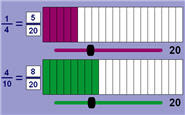## Monday, August 20, 2012

### Comparing Fractions

My fourth grade students are learning how to compare fractions. They've mastered this concept for very specific types of comparison problems, for example, when the denominators are the same and when the numerator is 1. Now I'm trying to teach them how to decide if a fraction is less than or greater than 1/2. They seemed to really grasp the idea that there are many ways to express the quantity 1/2 using fractions - 2/4, 3/6, 4/8, etc. The students recognized that the denominator is always double the numerator. In fact, one particularly clever student suggested the fraction 1/8 / 1/4, remembering the relative sizes of the fraction pieces we had used during the beginning of the unit. Despite this, my students are having difficulty determining whether a fraction such as 3/7 is more or less than 1/2.To help students visualize more difficult fractions, I designed a simple mathlet that draws two partitioned rectangles whose shaded sections represent the fractions typed in by the students. My students set one fraction to 1/2 and then alter the other fraction for comparison. I think they are starting to develop a better sense of relative sizes among fractions. Feel free to try it with your own students.